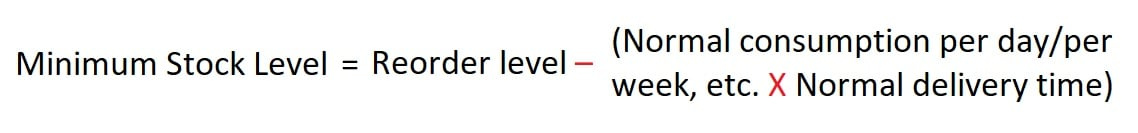# Minimum Stock Level or Minimum Stock Limit### Written byTrue Tamplin, BSc, CEPF®

Updated on July 22, 2022

## Minimum Stock Level: Definition and Explanation

A minimum stock level is a threshold value that indicates the level below which actual material stock items should not normally be allowed to fall.

In other words, a minimum stock level is a minimum quantity of a particular item of material that must be kept at all times.

The fixing of this level acts as a safety measure. For this reason, the minimum stock level is commonly known as safety stock or buffer stock.

If the actual stock falls below the minimum stock level, there is a risk of stopping production. Furthermore, management must prioritize the acquisition of fresh supplies.

The main objective of fixing the minimum stock level, and thus the minimum level of materials, is to ensure that the required quantity of each item is available at all times.

### Main Factors Involved in Fixing the Minimum Stock Level

The main factors to consider when fixing the minimum stock level are:

• Average rate of consumption of materials
• Time needed to procure fresh supplies under top priority conditions
• Reorder level
• Production requirements in terms of materials
• Minimum quantity of materials that can be procured advantageously

### Formula for Minimum Stock Level

The minimum stock level can be calculated by applying the following formula:## Examples

### Example 1

Suppose we have the following information:

• Normal consumption = 300 units per week
• Normal delivery time = 7 weeks
• Reorder level = 2,400 units

Calculate the minimum stock level.

### Solution 1

Based on this information, the minimum stock level can be calculated as follows:Minimum Stock Level=  2,400 - (300 x 7) = 300 units

### Example 2

In a manufacturing operation, a particular material is used as follows:

• Maximum consumption = 9,000 units per week
• Minimum consumption = 3,000 units per week
• Normal consumption = 6,000 units
• Reorder level = 36,000 units
• Time required for delivery = 4 to 6 weeks
• Time required for emergent supplies: 1 week

Calculate the minimum stock level.

### Solution 2

Since we know the above mentioned formula for calculating the minimum stock level, let’s start by calculating the reorder level first.

Reorder Level = Maximum Consumption per week x Maximum time required to obtain suppliers = 9,000 units x 6 weeks = 54,000 units

As the next step, we can calculate minimum stock level as follows:Minimum Stock Level = 54,000 units - (6,000 units x 5 weeks) = 54,000 - 30,000 = 24,000 units

Note: The average time required to obtain suppliers was calculated in the following way:

= (Minimum period + Maximum Period) / 2 = 4 weeks + 6 weeks / 2 = 5 weeks

Finance Strategists teams up with only the best financial firms in the nation to provide you with trusted financial advisors. Reach out to a financial advisor in Russellville, AR or visit our financial advisor page if you live outside the area.

## Minimum Stock Level or Minimum Stock Limit FAQs